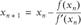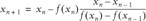# Using Classical Methods for Solving Equations

Problem

You've seen how to leverage Excel's built-in features to solve linear and nonlinear equations and systems, but you'd still like to know how to go about implementing some classical equation-solving algorithms in Excel.

Solution

You can use VBA to program any algorithm, just as you might in other programming languages. In the discussion to follow, I'll show you how to implement two classical methods in VBA: Newton's method and the secant method.

Discussion

The examples in this recipe will show how you might implement Newton's method and the secant method. These are well-known methods and are treated in most advanced mathematics and numerical methods texts. Further, you can find implementations of these methods in various languages, such as Fortran and C, on the Web. It's not a great leap to implement these methods in VBA in the form of a custom function, which you can then call from an Excel spreadsheet.

For the examples in this recipe, let's reconsider the cubic polynomial equation already discussed in Recipe 9.1. The equation is repeated here for convenience:A quick plot of this equation, as shown earlier in Figure 9-6, shows about where these three roots lie. You could easily use Solver to accurately find each of these roots. Or, you could use Newton's method or the secant method (or any of a number of other methods you could program), as discussed in the remainder of this recipe.

Newton's method

Newton's method is based on the idea of estimating values of x that are roots of the equation by taking the intersection of a line tangent to the curve under consideration at some assumed starting x-value. Subsequent estimates are made repeatedly using this approach until the estimate x-value converges on the true root. The basic formula for predicting each new x-value as you approach a root is:Notice that in Newton's method you must be able to compute, or estimate, the first derivative of the curve under consideration. In the example considered here, we have an equation that allows you to explicitly compute the derivative. In other cases where you can't easily compute the derivative, or where it is impossible to do so explicitly (such as when part of your function relies on tabular data), you have to resort to numerical derivatives. Recall from the introduction to this chapter that Solver uses numerical derivatives and allows you to select either forward differencing or central differencing (refer to Recipe 10.6 for a discussion of these and other techniques).

The structure of the above equation should give you some hints as to how you might implement Newton's method. First, we need a subroutine to evaluate the function under consideration. Second, we need another subroutine to evaluate the derivative of that function. Third, we need a subroutine that will iteratively apply the above equation starting from some initial guess until it converges on a root. I've prepared just such subroutines for this example. The first one is shown in Example 9-5 and it is used to evaluate the function under consideration at some given x-value.

Example 9-5. Function Fx

 ```Public Function Fx(x As Double) As Double Fx = 1 + x * (3 + x * (3 * x - 7)) End Function ```

This subroutine is actually a Function, and therefore it returns a value to the calling subroutine. In this case, it returns the value of the cubic equation we're considering for this example. (If you were dealing with some other equation, you would change the function Fx accordingly.)

The next subroutine, shown in Example 9-6, is a Function that returns the derivative of our cubic equation for some given value of x.

Example 9-6. Function dFx

 ```Public Function dFx(x As Double) As Double dFx = 3 + x * (9 * x - 14) End Function ```

The final required subroutine is shown in Example 9-7 and actually implements Newton's method, making periodic calls to Fx and dFx.

Example 9-7. Newton's method

 ```Public Function NewtonsMethod(x0 As Double, e As Double, n As Integer) As Double Dim i As Integer Dim err As Double Dim xn As Double Dim xn1 As Double i = 0 err = 9999 xn = x0 While (err > e) And (i < n) xn1 = xn - Fx(xn) / dFx(xn) err = Abs(xn1 - xn) xn = xn1 Wend NewtonsMethod = xn End Function ```

Notice again that this subroutine, NewtonsMethod, is actually a Function. This means you'll be able to call it directly in a spreadsheet cell formula and it will return a result in the calling cell. I'll show you how shortly, but first I want to go through the function NewtonsMethod.

NewtonsMethod takes three parameters. The first one, x0, serves as your initial guess at a root. The second parameter, e, is a convergence tolerance, which should be a small real number (e.g., 0.001). e is used to determine when the function has converged on a possible root. The third and final parameter, n, is an iteration limit. It is used to prevent the function from iterating indefinitely in the event it can't converge on a root.

Several variables are declared upon entering the function. These include i, which is just a counter variable; err, which is used to store the difference between successive estimates for x; xn, which is used to store estimate xn; and xn1, which is used to store estimate xn+1.

Next, these variables are initialized. i is set to 0. err is set to some large number. And xn is set to the initial guess x0.

The While loop is where Newton's method is applied. The While loop will iterate until the computed error, err, is less than the convergence tolerance, e, or until the maximum number of iterations, n, has been reached.

A few things take place within the While loop. First, Newton's formula is applied, calling Fx and dFx to estimate the next x-value given the current estimate. Then the error is computed. Finally, the current x-estimate is updated before starting the next iteration.

When the While loop ends due either to converging on a root or reaching the iteration limit, the current estimate for x is returned by the function.

To call this function from a spreadsheet cell, you could enter something like =NewtonsMethod(0, 0.0001, 100) in any given cell. Excel will automatically call NewtonsMethod every time the spreadsheet needs updating (there's no need to use a button control, because NewtonsMethod is a Function). In this case, the cell will contain the value -0.215, which is indeed a root to the equation under consideration. Notice the initial guess I used here of 0.

To capture the other roots, you could change your initial guess. Changing the initial guess to 0.5 yields the root at x = 1.0. Again, changing the initial guess to 1.75 yields the root at x = 1.549.

Secant method

An alternative to Newton's method is the secant method . The secant method has the advantage that you do not need to compute the derivative of the equation under consideration. As a trade-off, you do have to supply two initial guesses so that a secant line can be constructed instead of a tangent line for making x predictions. The formula for estimating xn+1 using the secant method is:To implement the secant method, we still need the function Fx as shown in Example 9-5, but we do not need the function dFx.

The VBA Function that implements the secant method for this example is shown in Example 9-8.

Example 9-8. Secant method

 ```Public Function SecantMethod(x0 As Double, x1 As Double, e As Double, n As Integer) As Double Dim i As Integer Dim err As Double Dim xn As Double Dim xn1 As Double Dim xnm1 As Double i = 0 err = 9999 xn = x1 xnm1 = x0 While (err > e) And (i < n) xn1 = xn - Fx(xn) * (xn - xnm1) / (Fx(xn) - Fx(xnm1)) err = Abs(xn1 - xn) xnm1 = xn xn = xn1 Wend SecantMethod = xn End Function ```

This function is substantially similar to the function NewtonsMethod. There are a few differences that I'll highlight. First, notice the additional parameter, x1, which represents the second initial guess for x. Remember that for the secant method we need two initial guesses for x.

The remaining differences are found within the While loop. As you can see, the expression for predicting xn1 has been replaced with the secant formula. And each iteration requires updating both xn and xn-1, which are stored in the variables xn and xnm1, respectively.

You can use SecantMethod in a cell formula just as you did NewtonsMethod. For example, I entered the following three formulas in three different cells in a spreadsheet:

``` =secantmethod(0, -0.1, 0.0001, 100)
=secantmethod(0.5, 0.6, 0.0001, 100)
=secantmethod(1.75, 1.7, 0.0001, 100)
```

These three formulas returned the values -0.215, 1.000, and 1.549, respectively. As you see, these roots are identical to those obtained using Newton's method, and in both cases the results agree well with what you can glean by examining the plot of the example cubic equation in Figure 9-6.Excel Scientific and Engineering Cookbook (Cookbooks (OReilly))
ISBN: 0596008791
EAN: 2147483647
Year: N/A
Pages: 206
Authors: David M Bourg